Next: Hydrostatic equilibrium of the Up: Classical thermodynamics Previous: Calculation of specific heats

Suppose that the temperature of an ideal gas is held constant by keeping the gas in thermal contact with a heat reservoir. If the gas is allowed to expand quasi-statically under these so called isothermal conditions then the ideal equation of state tells us that(311)

This is usually called the isothermal gas law.

Suppose, now, that the gas is thermally isolated from its surroundings. If the gas is allowed to expand quasi-statically under these so called adiabatic conditions then it does work on its environment, and, hence, its internal energy is reduced, and its temperature changes. Let us work out the relationship between the pressure and volume of the gas during adiabatic expansion.

According to the first law of thermodynamics,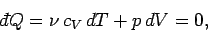(312)

in an adiabatic process (in which no heat is absorbed). The ideal gas equation of state can be differentiated, yielding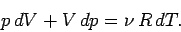(313)

The temperature increment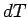can be eliminated between the above two expressions to give(314)

which reduces to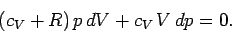(315)

Dividing through by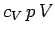yields(316)

where(317)

It turns out that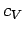is a very slowly varying function of temperature in most gases. So, it is always a fairly good approximation to treat the ratio of specific heats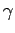as a constant, at least over a limited temperature range. Ifis constant then we can integrate Eq. (316) to give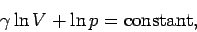(318)

or(319)

This is the famous adiabatic gas law. It is very easy to obtain similar relationships betweenandandandduring adiabatic expansion or contraction. Since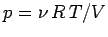, the above formula also implies that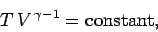(320)

and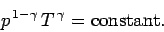(321)

Equations (319)-(321) are all completely equivalent.Next: Hydrostatic equilibrium of the Up: Classical thermodynamics Previous: Calculation of specific heats
Richard Fitzpatrick 2006-02-02